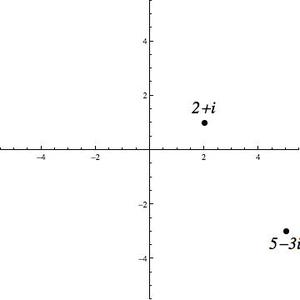# Complex Distance

Alignments to Content Standards: N-CN.B.6

In this problem, you will compute some values related to the complex numbers $2+i$ and $5-3i$.

1. Plot $2+i$ and $5-3i$ in the complex plane.
2. How far is $2+i$ from $5-3i$? That is, what is the length of the line segment between $2+i$ from $5-3i$?
3. What is the modulus of the difference of $2+i$ and $5-3i$?
4. What is the midpoint of the line segment between $2+i$ and $5-3i$?
5. What is the average of $2+i$ and $5-3i$?
6. Describe, using the complex plane, the relationship between your answers in (b) and (c).
7. Describe, using the complex plane, the relationship between your answers in (d) and (e).

## IM Commentary

This problem is intended to reinforce the geometric interpretation of distance between complex numbers and midpoints as modulus of the difference and average respectively.

Using the image of the two points in the plane will help solidify these concepts.

A right triangle with vertices at $2+i$ and $5-3i$ and $2-3i$ may help the teacher explain how to compute the distance in (b).

For part (e), the teacher may need to remind the class that the average of two real numbers $x$ and $y$ is $\frac12(x+y)$ and that this formula can be applied to complex numbers. Likewise a comparison to the average of real numbers in (g) can be helpful. Students might build on the observation that the average of two real numbers is also the midpoint of the line segment between those real numbers (on the number line or in the plane).

Parts (f) and (g) are optional and do not directly reflect the standard. Rather, these questions open student discussion on average, midpoint, length and modulus. The use of the verb "describe" rather than "explain" in these two problems is deliberate. Discussion should focus on using common and personal language rather than a formal argument in response to these questions.

Parts (f) and (g) are also an opportunity to emphasize connections to mathematical practices 2 (reason abstractly and quantitatively) and 3 (construct viable arguments).

The teacher may want to give several other pairs of complex numbers prior to staring parts (f) and (g). Going through steps (a) through (e) with the pair 4 and $2i$ as well as the pair $2+3i$ and $2-i$ may help illuminate these relationships for students.

## Solution

1.2. As points, the complex numbers are $(2,1)$ and $(5,-3)$. The distance formula yields $\sqrt{(2-5)^2+(1-(-3))^2} = \sqrt{3^2+4^2} = 5$.
3. The modulus of $(2+i)-(5-3i)$ is $|-3+4i| = 5$.
4. As points, the complex numbers are $(2,1)$ and $(5,-3)$. The midpoint is $((2+5)/2, (1-3)/2) = \left(\frac72, -1\right)$ or $\frac72-i$ or $3\frac12-i$.
5. The average of the complex numbers are $\frac12((2+i)+(5-3i)) = \frac72-i$ or $3\frac12-i$.
6. Students should point out that the geometric and modular interpretations in (b) and (c) are the same.
7. Students should point out that the midpoint and average are the same.